NEET  >  Test: Heat

# Test: Heat - NEET

Test Description

## 30 Questions MCQ Test Physics Class 11 - Test: Heat

Test: Heat for NEET 2023 is part of Physics Class 11 preparation. The Test: Heat questions and answers have been prepared according to the NEET exam syllabus.The Test: Heat MCQs are made for NEET 2023 Exam. Find important definitions, questions, notes, meanings, examples, exercises, MCQs and online tests for Test: Heat below.
Solutions of Test: Heat questions in English are available as part of our Physics Class 11 for NEET & Test: Heat solutions in Hindi for Physics Class 11 course. Download more important topics, notes, lectures and mock test series for NEET Exam by signing up for free. Attempt Test: Heat | 30 questions in 60 minutes | Mock test for NEET preparation | Free important questions MCQ to study Physics Class 11 for NEET Exam | Download free PDF with solutions
 1 Crore+ students have signed up on EduRev. Have you?
Test: Heat - Question 1

### The compounds C2H5OC2H5 and CH3OCH2CH2CH3 are

Detailed Solution for Test: Heat - Question 1

Here, ether is functional group and whenever there is change around same functional group they are metamers to each other.
These are chain as well as metamers but priority is given to metamerism even if we have to choose between metamer and position then, again metamer is to be opted first.

Test: Heat - Question 2

### The number of isomers of pentane is

Detailed Solution for Test: Heat - Question 2

Pentane (C5H12) is an organic compound with five carbon atoms. Pentane has three structural isomers that are n-pentane, Iso-pentane (methyl butane) and neopentane (dimethylpropane).

Test: Heat - Question 3

### How many minimum no. of C-atoms are required for position & geometrical isomerism in alkene?

Detailed Solution for Test: Heat - Question 3

The smallest alkene showing geometrical isomerism is but−2−ene (4−C atoms)
The smallest alkene showing position isomerism is butene (4−C atoms), where the position of double bonds may vary.

Test: Heat - Question 4

How many structural formula are possible when one of the hydrogen is replaced by a chlorine atom in anthracene?

Detailed Solution for Test: Heat - Question 4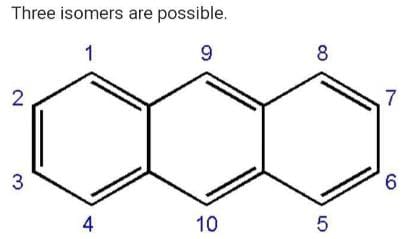Position 1,4,5,8 are equal.
Similarly 2,3,6,7 are equal.
Position 9 and 10 are equal.
So we will get three possible isomers

Test: Heat - Question 5

Which of the following connot be written in an isomeric form?

Detailed Solution for Test: Heat - Question 5

CH3​−CH(OH)−CH2​−CH3​ can have position isomer.
CH3​−CHO can have functional isomers (enol).
Cl−CH2​CH2​−Cl can have position isomer.
But CH2​=CH−Cl has one isomer but it is not preferred IUPAC name.

Test: Heat - Question 6

The number of cis-trans isomer possible for the following compound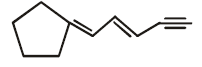Detailed Solution for Test: Heat - Question 6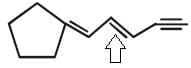Since only this is a double bond with different groups attached so only it will show cis-trans isomerism

Test: Heat - Question 7

The number of isomers of dibromoderivative of an alkene (molear mass 186 g mol–1) is

Detailed Solution for Test: Heat - Question 7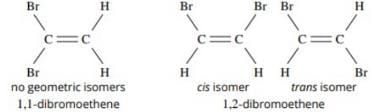Test: Heat - Question 8

Increasing order of stability among the three main conformation (i.e. eclipse, anti, gauche) of ethylene glycol is:

Detailed Solution for Test: Heat - Question 8

In the anti conformation the atoms are far apart ergo causing minimum repulsion whereas the opposite is true for eclipsed conformation. Gauche is in an in between stage.

Test: Heat - Question 9

How many primary amines are possible for the formula C4H11N?

Detailed Solution for Test: Heat - Question 9

The following 4 structures are possible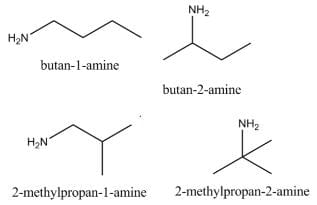Test: Heat - Question 10

The R/S configuration of these compounds are respectively.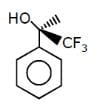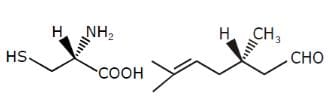Detailed Solution for Test: Heat - Question 10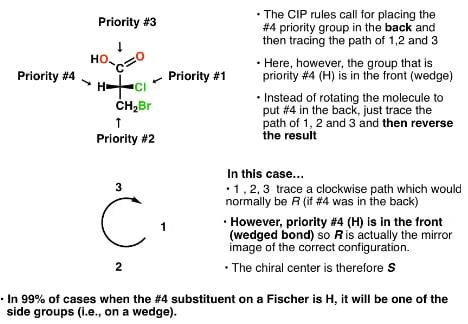Applying the previously stated results we get A as the answer

Test: Heat - Question 11

How many planes (pos) are presents in Anthracene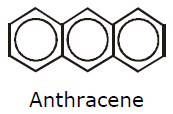Detailed Solution for Test: Heat - Question 11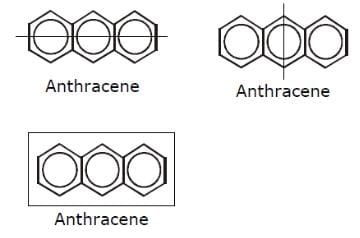Test: Heat - Question 12

Two neighbours of homologous series differ by

Detailed Solution for Test: Heat - Question 12

A homologous series is a series of compounds in which each member differs from the next/previous by CH2 or 14 mass units. Physical properties change in a homologous series but chemical properties remain almost the same because the functional group does not change.

Test: Heat - Question 13

Geometrical isomers can be

Detailed Solution for Test: Heat - Question 13

Diastereomers are isomers which are not mirror images of each other, while enantiomers are those which are mirror images of each other. A geometric isomer without any chiral centre is a mirror image of itself. Also, 2 geometric isomers, which are not mirror images of each other will be diastereomers.
Hence, geometrical isomers can be diastereomers or enantiomers.

Test: Heat - Question 14

Phenol and benzyl alcohol are

Detailed Solution for Test: Heat - Question 14

Phenol is C6​H5​(OH) while benzyl alcohol is C6​H5​(CH2​OH).
Here there is a difference of −HC2​ between their molecular formula.
According to the definition of homologous series, the adjacent members of the series differ by −CH2​ group.
Thus, Phenol and benzyl alcohol are homologous of each other.

Test: Heat - Question 15

C3H8 belongs to the homologous series of

Detailed Solution for Test: Heat - Question 15

C3H6 and C4H8 belongs to same homologous series as they both belongs to alkene series whose formula is CnH2n, here n = no. of carbon atoms.

Test: Heat - Question 16

Which of the follownig compounds is (S)–4-chloro-1-methylcyclohexene ?

Detailed Solution for Test: Heat - Question 16

Because when you see the question there is 1-methylcyclohexene this means the first numbering goes to methyl group and 'ene' group that is double bond and then it goes to 4 chloro that means from up to down including double bond we have to take chlorine on 4th position and it is (s) that means it is above the surface.

Test: Heat - Question 17

Which of the following compounds has two stereogenic centers (asymmetric carbons) ?

Detailed Solution for Test: Heat - Question 17

Asymmetric carbon is the carbon which has all the four attached groups different.And these carbons are also called chiral carbons.
Hence option A has asymmetric or chiral carbon.
Hence option A is correct.

Test: Heat - Question 18

Which of the following structures represents a chiral compound ?

Detailed Solution for Test: Heat - Question 18

This compound is chiral because its molecule has two chiral carbon atoms (C−3 and C-4).
ether molecules are chiral due to the presence of a plane of symmetry.

Test: Heat - Question 19

Examine the compound on the right. How many stereoisomers having this constitution are possible ?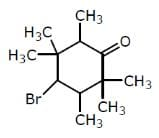Detailed Solution for Test: Heat - Question 19

Explanation : 3 chiral centres

Number of Stereoisomers = 2n

= 23

= 8

Test: Heat - Question 20

Which of the following heptanols are chiral 1-heptanol, 2-heptanol, 3-heptanol, 4-heptanol.

Detailed Solution for Test: Heat - Question 20

As you can see, the third carbon atom from the left has three different groups attached to it namely 1. hydroxyl groups attached to namely 1. hydroxyl,
2. ethyl
3. n-butyl,
4. hydrogen
Hence the mentioned carbon atom is a chiral centre. Now any molecule having one chiral centre is a chiral molecule, i.e. 2 forms namely (R) and (S) forms of the molecule exist.
Please note that if the molecule has more than one chiral centre, We cannot directly conclude that the molecule will be chiral.

Test: Heat - Question 21

Which of the following will exhibit geometrical isomerism ?

Detailed Solution for Test: Heat - Question 21

1-phenyl-2-butene shows geometrical isomerism in the form of cis and trans isomers.
However, the geometrical isomerism is not possible for 3-phenyl-1-butene, 2-phenyl-1-butene and 1,1-diphenyl-1-propane.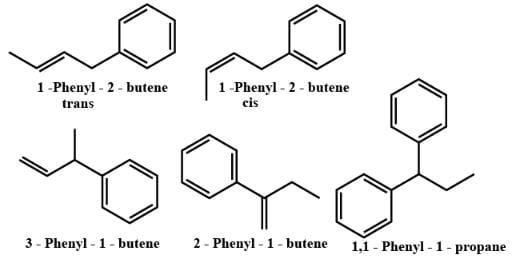Test: Heat - Question 22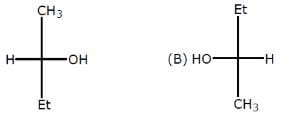Relation between given pair is

Detailed Solution for Test: Heat - Question 22

The above pairs are known as identical pair. An isomer of a molecule has the number of atoms of each element but has a  different arrangement of the atoms.It has  the same molecular  formula as the other molecule , with a different chemical structure.

Test: Heat - Question 23

Following eclipsed form of propane is repeated after rotation of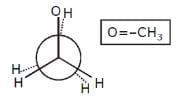Test: Heat - Question 24

Stereoisomers differ from each other in what respect ?

Detailed Solution for Test: Heat - Question 24

Stereoisomers. Generally defined, stereoisomers are isomers that have the same composition (that is, the same parts) but that differ in the orientation of those parts in space.

Test: Heat - Question 25

The number of isomers of C5H10 is

Detailed Solution for Test: Heat - Question 25

Explanation : Given molecular formula is C5H10.

⇒ There are 6 isomeric alkenes by including Cis/trans isomers of pent−2−ene.

⇒ 7 Cycloalkanes also possible.

Totally it have 13 isomers.

Test: Heat - Question 26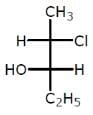The compound with the above configuration is called.

Detailed Solution for Test: Heat - Question 26

4th priority group always lie in vertical
Clockwise: R
Anticlockwise: S
At 2nd carbon 1 → 2 → 3 configuration is r but 4 groups are horizontal so conf. change from R to s
At 3rd carbon a → b → c conf. is r but d group is horizontal so conf. change from R to S
Hence,
2s,3s)-c-chloro-3 hydropentane

Test: Heat - Question 27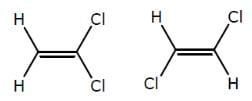The above compounds differ in

Detailed Solution for Test: Heat - Question 27

The first compound is in cis phase and the other one is trans phase. they differ in their structure.

Test: Heat - Question 28

The compound C2H5OC2H5 and CH3OCH2CH2CH3 are

Detailed Solution for Test: Heat - Question 28

The compounds C2​H5​OC2​H5​ and CH3​OCH2​CH2​CH3​ are metamers.
They differ in the nature of the alkyl groups.

Test: Heat - Question 29

Which conformer of cyclohexane is chiral

Detailed Solution for Test: Heat - Question 29

We know that if a compound is symmetrical then then it is not chiral. hence chair conformation and boat conformations. Both are achiral whereas twist boat is chiral.

Test: Heat - Question 30

Minimum C atoms required for a compound to show geometrical isomerism :

Detailed Solution for Test: Heat - Question 30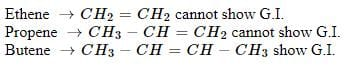## Physics Class 11

130 videos|483 docs|210 tests(Scan QR code)